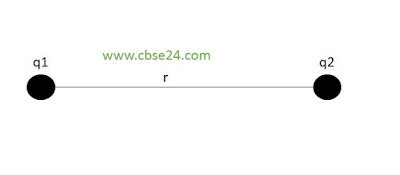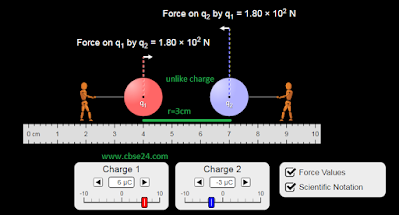# Coulomb's Law of Electric Force | cbse24.com

## In 1785 the French physicist Charle Augustin Coulomb experimentally measured the electric forces between small charged spheres by using a torsion balance. He formulated his observation in the form of coulomb's law which is the electrical analogue of Newton's of Universal Gravitation in mechanics.

Coulomb's law states that the force of attraction or repulsion between two stationary point charges (i)directly proportional to the product of the magnitude of the two charges (ii) inversely proportional to the square of the distance between the. This force acts the line joining the two charges.Coulomb's law

If two-point charges q1 and q2 are separated by distance r, then the force F of attraction or repulsion between them is such that

Fq1q2     and   F1r2

Fq1q2r2orF=kq1q2r2

Where k is constant of proportionality called electrostatic force constant. The value of k depends on the nature of the medium between the two charges and the system if units chosen to measure F,q1,q2, and r.

For the two charges located in free space and SI units we have

k=14πϵ0=9×109Nm2C2

where εo is called the permittivity of free space. So we can express Coulomb's law in SI units as

F=14πϵ0.q1q2r2

## Unit of charge:

(i) The SI unit of charge is the coulomb. In the above equation if q1=q2=1C and r=1m, then

F=14πϵ0=9×109N

So one coulomb is that amount of charge that repels an equal and similar charge with a force of 9 x 10^{9}N  when placed in vacuum at a distance of one meter from it.

(ii) In electrostatics, CGS system the unit of charge is known as electrostatics unit of charge(E.S.U) or stat coulomb(stat C)
one E.S.U of charge or one state coulomb is that charge which repels an identical charge in vacuum at a distance of one centimeter from it with a force of 1 dyne.

1coulomb=3 x 10 ^{9}stat coulomb
= 3 x 10 ^{9}E.S.U of charge

(iii) In electromagnetic CGS system, the unit of charge is abcoulomb or electromagnetic unit of charge(E.M.U of charge)

1 coulomb=1/10 abcoulomb=1/10e.m.u of charge

## Knowledge Point:

• When the linear sizes of charged bodies are much smaller than the distance between them, their sizes may be ignored and the charged bodies are called point charges.
• Coulomb's law is valid only for point charges.
• In the SI unit the exact value of the combination 4πεo=(10^7/c^2 )C^{2}N^{-1}m^{-2} (where c is the speed of light
• Electrostatic force constant k=8.98755 x 10^{-12} Nm^{2}C{-2} ≃ 9 x 10 ^9 Nm^{2}C^{-2}
• The permittivity of free space εo=8.8551485 x 10^-12 C^{2}N^{-1}m^{-2}

Coulomb's law in vector form:

(a) For like charge:

As shown in fig consider two positive point charges q1 and q2  placed in vacuum at distance r from each other. They repel each other.Repulsive Coulomb forces for q1q2>0Simulation of Coulomb force due to like charge

In vector form, coulomb's law may be expressed as

F21=Force.on.charge,q2.and.q1

=14πϵ0.q1q2r2r^12

Where r^12=r12r  is a unit vector in the direction from q1 to q2.
Similarly, F12=Force on charge q1 due to q2

=14πϵ0.q1q2r2r^21

Where r^21=r21r  is a unit vector in the direction from q2 to q1.

(b)For unlike charge:

The Colombian force between unlike charges(q1q2<0) is attractive as shown in fig.Attractive coulomb force for q1 q2<0Simulation of Coulomb force due to unlike charge

### Importance of vector form:

 As,r^21=r^12,therefore,F21=F12
This mean that the two charges exert equal and opposite force on each other. So Colombian forces obey Newton's third law of motion.
 As the Colombian force act along the line joining the centers of two charges so they are central forces.

*